1593083880

Linear Algebra from Strang.

Professor Strang’s — Introduction to Linear Algebra — is an incredible journey into the hart of Linear Algebra. Over the next few articles I aim to illuminate some of the sections in the light of Python. Enjoy!

In this article I want to look into a special class of matrices, _projection matrices. _First looking at some fairly intuitive projection matrices that project lines in 3D onto the orthonormal basis they are defined in. Secondly we will look at how you can project any arbitrary vector onto a line. We will then finish off by generalising to how we can define a projection matrix for any plane.

All the way along we will translate these ideas into the language of python using vanilla numpy and plotly for the visuals.

A note before you start. You should have an understanding of basic matrix operations before reading this article, for example inner and outer products.

Projection matrices

Given some n dimensional vector, v = (a1, a2, …, an) we can consider projections of this vector onto various subspaces in Rn. We describe these projections with, you guessed it, projection matrices.

Let’s restrict ourselves to three dimensions. Then we can describe the projection matrices that project a vector v onto the z axis and xy plane respectively.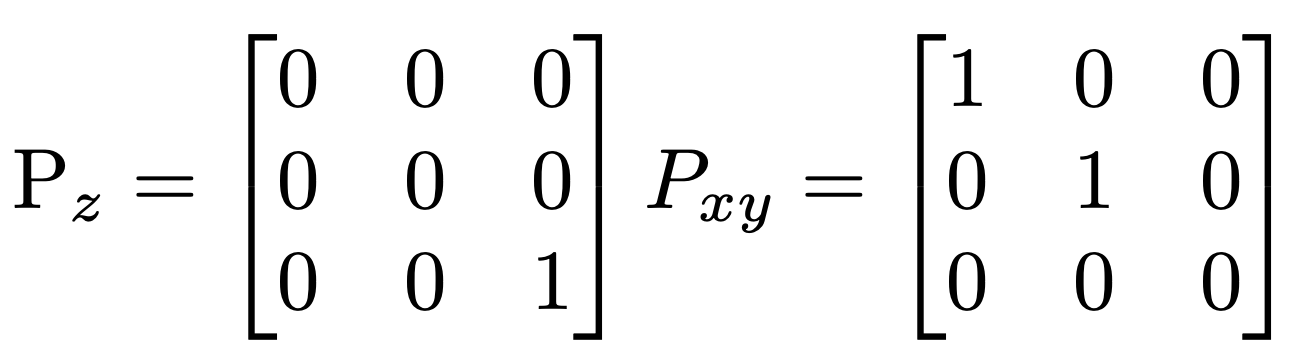Projection matrices. When acting on a 3 dimensional column vector they pick out the components in the z and xy plane respectively.

Lets say we have some vector v then we can project this matrix onto the z axis like so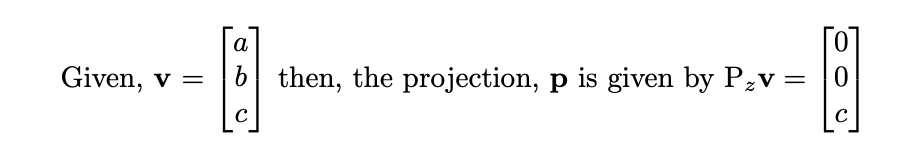We can see that the projection matrix picks out the components of v that point in the plane/line we wish to project onto. Strang describes the purpose of a projection matrix as follows.

#python #mathematics #matrix #linear-algebra #data-science

Buddha Community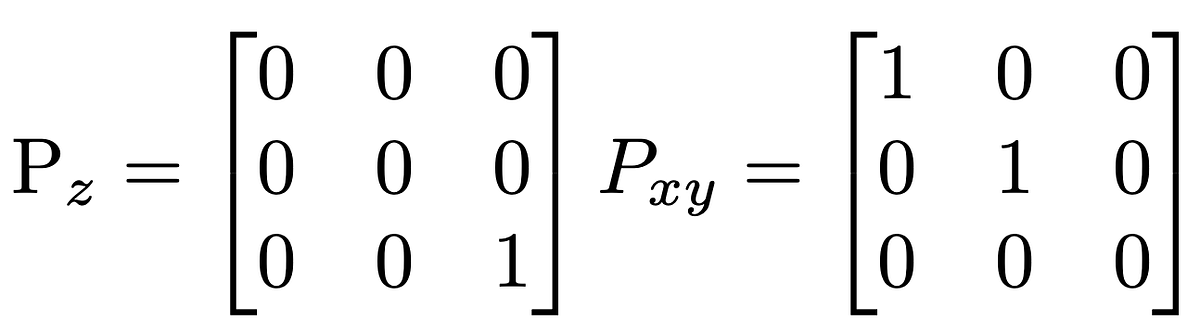1624447260

Course Review: Python for Linear Algebra

Because I am continuously endeavouring to improve my knowledge and skill of the Python programming language, I decided to take some free courses in an attempt to improve upon my knowledge base. I found one such course on linear algebra, which I found on YouTube. I decided to watch the video and undertake the course work because it focused on the Python programming language, something that I wanted to improve my skill in. Youtube video this course review was taken from:- (4) Python for linear algebra (for absolute beginners) — YouTube

The course is for absolute beginners, which is good because I have never studied linear algebra and had no idea what the terms I would be working with were.

Linear algebra is the branch of mathematics concerning linear equations, such as linear maps and their representations in vector spaces and through matrices. Linear algebra is central to almost all areas of mathematics.

Whilst studying linear algebra, I have learned a few topics that I had not previously known. For example:-

A scalar is simply a number, being an integer or a float. Scalers are convenient in applications that don’t need to be concerned with all the ways that data can be represented in a computer.

A vector is a one dimensional array of numbers. The difference between a vector is that it is mutable, being known as dynamic arrays.

A matrix is similar to a two dimensional rectangular array of data stored in rows and columns. The data stored in the matrix can be strings, numbers, etcetera.

In addition to the basic components of linear algebra, being a scalar, vector and matrix, there are several ways the vectors and matrix can be manipulated to make it suitable for machine learning.

I used Google Colab to code the programming examples and the assignments that were given in the 1 hour 51 minute video. It took a while to get into writing the code of the various subjects that were studied because, as the video stated, it is a course for absolute beginners.

The two main libraries that were used for this course were numpy and matplotlib. Numpy is the library that is used to carry out algebraic operations and matplotlib is used to graphically plot the points that are created in the program.

#numpy #matplotlib #python #linear-algebra #course review: python for linear algebra #linear algebra1595377260

Linear Algebra: The hidden engine of machine learning

Algebra is firstly taken from a book, written by Khwarizmi(780-850 CE), which is about calculation and equations. It is a branch of mathematics in which letters are used instead of numbers. Each letter can represent a specific number in one place, and a completely different number in another. Notations and symbols are also used in algebra to show the relationship between numbers. I remember about 17 years ago when I was an ordinary student in applied mathematics(ordinary graduate today!), I was so curious about some research in algebra, done by Maryam Mirzakhani(1977–2017), at Harvard University about analogous counting problem. This science has evolved a lot throughout history and now includes many branches.

Elementary algebra includes basic operations on four main operations. After defining the signs by which fixed numbers and variables are separated, methods are used to solve the equations. A polynomial is an expression that is the sum of a finite number of non-zero terms, each term consisting of the product of a constant and a finite number of variables raised to whole number powers.

Abstract algebra or modern algebra is a group in the algebra family that studies advanced algebraic structures such as groups, rings, and fields. Algebraic structures, with their associated homomorphisms, form mathematical categories. Category theory is a formalism that allows a unified way for expressing properties and constructions that are similar for various structures. Abstract algebra is so popular and used in many fields of mathematics and engineering sciences. For instance, algebraic topology uses algebraic objects to study topologies. The Poincaré conjecture proved in 2003, asserts that the fundamental group of a manifold, which encodes information about connectedness, can be used to determine whether a manifold is a sphere or not. Algebraic number theory studies various number rings that generalize the set of integers.

I believe that the most influencing branch of algebra in other sciences is linear algebra. Let’s suppose that you went out for jogging, that can’t be easy these days with Covid-19 lockdown, and suddenly a beautiful flower catches all your attention. Please don’t be rush and pick it, just take a picture, others can enjoy it, as well. After a while when you look at this picture, you can recognize the flower in the image, because the human brain has evolved over millions of years and able to detect such a thing. We are unaware of the operations that take place in the background of our brains and enable us to recognize the colors in the image, they are trained to do this for us automatically. But, it’s not easy to do such a thing with a machine, that’s why this is one of the most active research areas in machine learning and deep learning. Actually, the fundamental question is: “How does a machine store this image?” You probably know that today’s computers are designed to process only two numbers, 0 and 1. Now, how can an image like this with different features be stored? This is done by storing the pixel intensity in a structure called a “matrix”.

The main topics in linear algebra are vectors and matrices. Vectors are geometric objects that have length and direction. For example, we can mention speed and force, both of which are vector quantities. Each vector is represented by an arrow whose length and direction indicate the size and direction of the vector.

The addition of two or more vectors can be done based on ease of use using parallelogram methods or the method of images in which each vector is decomposed into its components along the coordinate axes. A vector space is a collection of vectors, which may be added together and multiplied by scalars. Scalars generally can be picked up from any field but normally are real numbers.

#matrice #machine-learning #linear-algebra #algebra #deep-learning #deep learning1593083880

Linear Algebra from Strang.

Professor Strang’s — Introduction to Linear Algebra — is an incredible journey into the hart of Linear Algebra. Over the next few articles I aim to illuminate some of the sections in the light of Python. Enjoy!

In this article I want to look into a special class of matrices, _projection matrices. _First looking at some fairly intuitive projection matrices that project lines in 3D onto the orthonormal basis they are defined in. Secondly we will look at how you can project any arbitrary vector onto a line. We will then finish off by generalising to how we can define a projection matrix for any plane.

All the way along we will translate these ideas into the language of python using vanilla numpy and plotly for the visuals.

A note before you start. You should have an understanding of basic matrix operations before reading this article, for example inner and outer products.

Projection matrices

Given some n dimensional vector, v = (a1, a2, …, an) we can consider projections of this vector onto various subspaces in Rn. We describe these projections with, you guessed it, projection matrices.

Let’s restrict ourselves to three dimensions. Then we can describe the projection matrices that project a vector v onto the z axis and xy plane respectively.Projection matrices. When acting on a 3 dimensional column vector they pick out the components in the z and xy plane respectively.

Lets say we have some vector v then we can project this matrix onto the z axis like soWe can see that the projection matrix picks out the components of v that point in the plane/line we wish to project onto. Strang describes the purpose of a projection matrix as follows.

#python #mathematics #matrix #linear-algebra #data-science1596790020

Appendix: Linear Algebra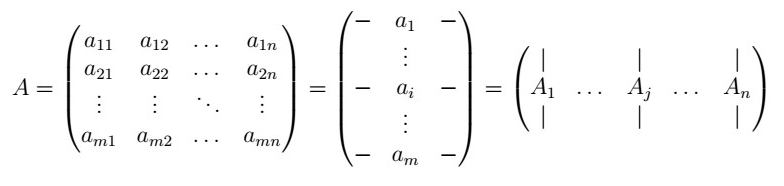These notes are intended to provide a “fast” collection of theoretical definitions, theorems, and concepts for Linear Algebra as a refresher only, and as an Appendix to my “A Complete Introduction to Time Series Analysis” series. If you are looking for a more comprehensive guide, please consider reading this CS229 Linear Algebra Review.

Groups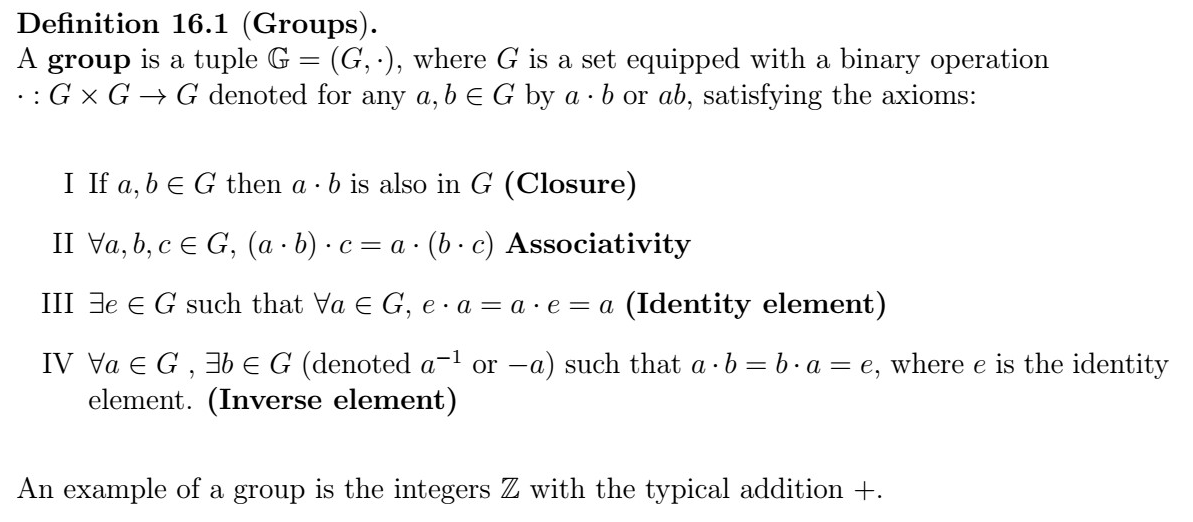Fields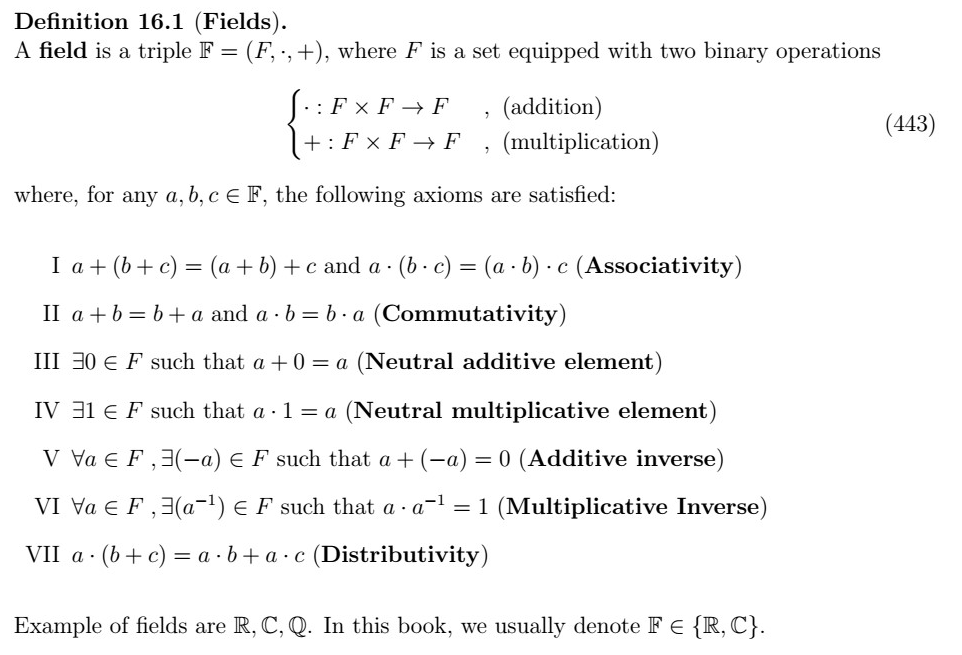Vectors and Matrices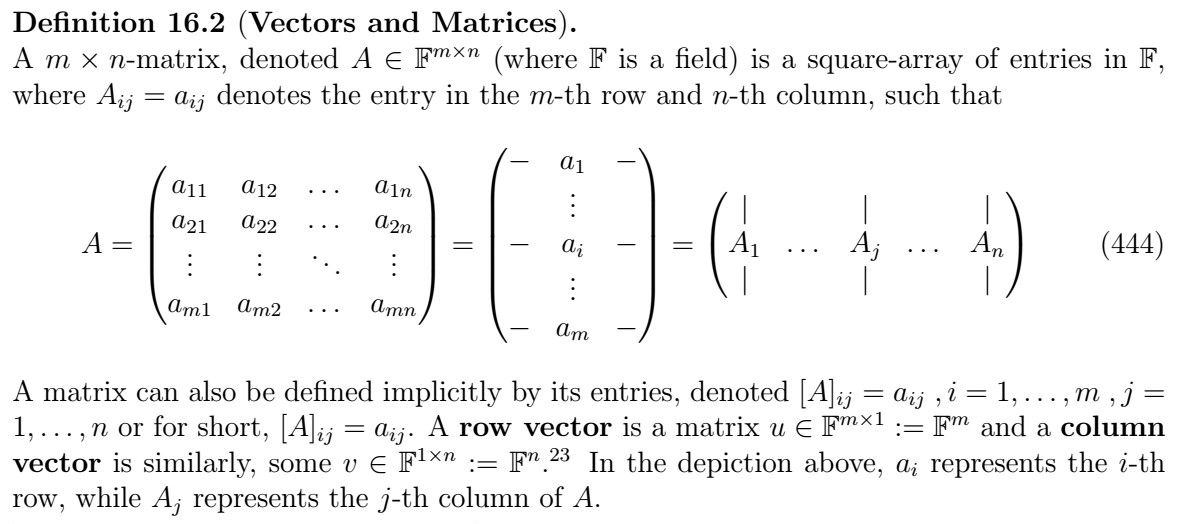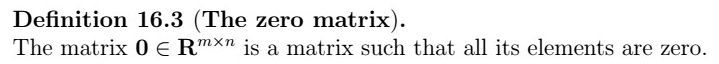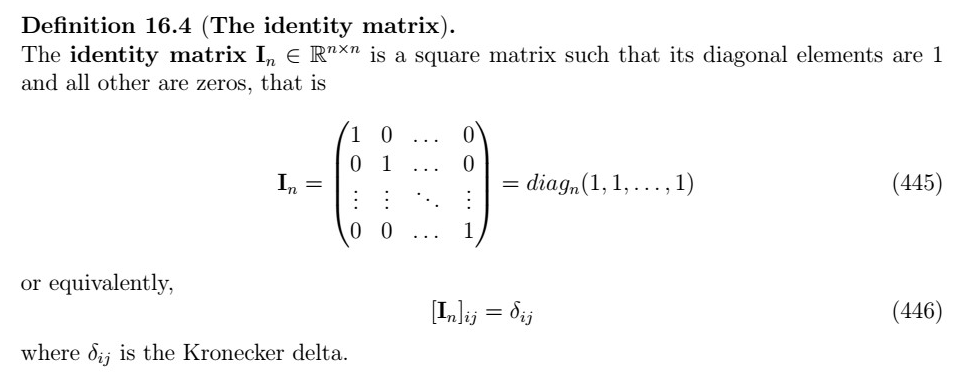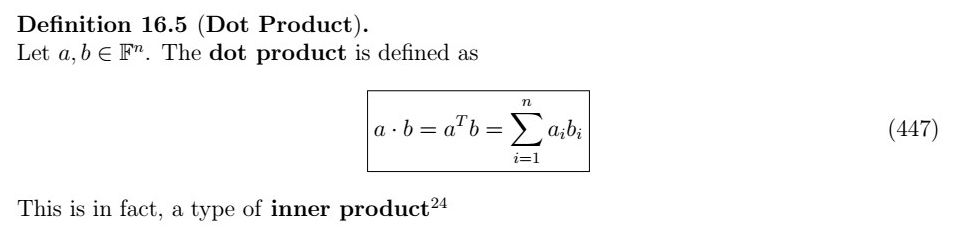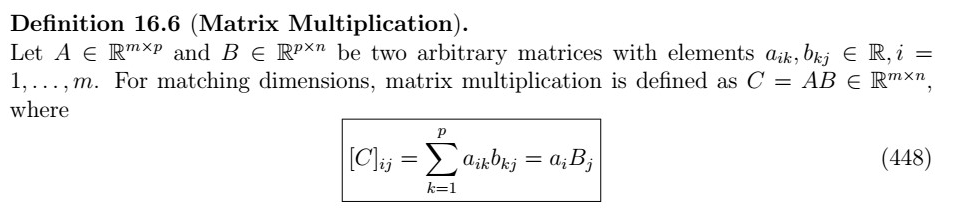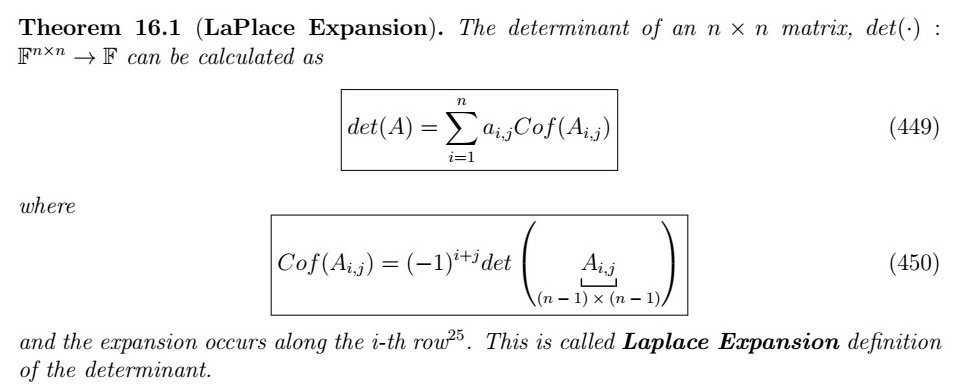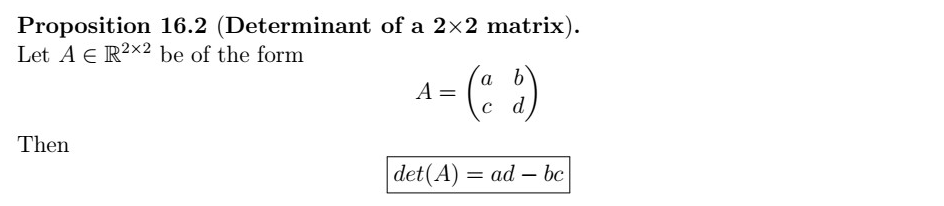#mathematics #statistics #machine-learning #time-series-analysis #linear-algebra #deep learning1604041200

Why Linear Algebra Is Important For Programming

The reasons might blow your mind.

Despite linear algebra is known for many use cases (especially in statistics), programmers might not know the reasons to learn linear algebra. Here, we’ll outline for reasons to learn linear algebra for programming.

1-Games are designed by the principle of linear and matrix transformations

You might say as a programmer “I don’t need to know linear algebra to create a cracking game. Calculations are being done by the computers anyway”. There’s truth to this statement even though it undermines the knowledge needed to create complex games. Especially when your game characters rotate, move from point A to point B, and all the features of the whole game itself.

2-Needed for a statistical programmingEven though computers do all the calculations for us. Still, they can’t interpret the results of our calculations whether they’re statistical or mathematical. So that’s why we need to know linear algebra if we want to do statistical programming. Especially in regression analysis, some topics of probability, operations research, mathematical statistics, and stochastics. When considered the use in a vast environment in statistics, interpreting the output and using it to make decisions.

3-Needed for mathematical programming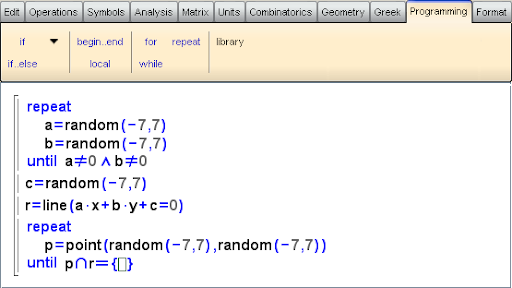Linear algebra is known for being a fundamental topic in mathematics that’s being used in every field of mathematics after calculus is learned. And nearly all the analysis in mathematics requires some sort of linear algebra when it comes to transformations, orthogonality, orthonormality, applied mathematics, and all sorts of analysis known and proven by scientific means.

4-Needed for advanced mathematical and statistical calculations

We simply have linear algebra mixed into our lives, calculations, and much other stuff that requires advanced calculations. I don’t even mention the fields in physics, biology, chemistry, engineering, economics, mathematics, and statistics. Especially when we consider the real-life applications of all these disciplines, linear algebra itself helps a lot when clearing the data and allowed R to be formed according to the logic of linear algebra.

What do you think about the relationship between linear algebra and programming? Share your thoughts in the comments section below.

#linear-algebra #statistics #coding #mathematics #programming